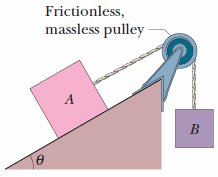# HW22#5 – Body A and body B. The coefficients of friction between A and the incline are…

Body A in the figure weighs 107 N, and body B weighs 35 N. The coefficients of friction between A and the incline are μs = 0.56 and μk = 0.25. Angle θ is 40°. Let the positive direction of an x axis be up the incline. Answer the following questions in unit-vector notation.(a) Find the acceleration of A if A is initially at rest.

(b) Find the acceleration of A if A is moving up the incline.

(c) Find the acceleration of A if A is moving down the incline.